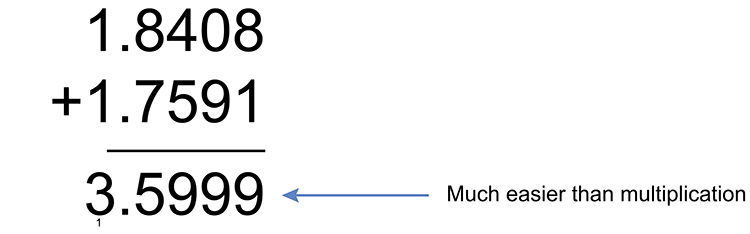# Logarithms - multiplication

Mathematicians found an easier way.

From our knowledge of indices.

a^m\timesa^n = a^(m+n)

or 10^2\times10^2 = 10^4

(10\times10)\times (10\times10) = (10\times10\times10\times10)

Here is the example again

69.31\times 57.43

This is the same as 10^(1.8408)\times 10^(1.7591) (see next page for explanation)This equals 10^(3.5999)

And 10^(3.5999) = 10^3\times 10^(0.5999)

= 1000\times 3.980

= 3980

(see next sections for explanation)